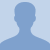# Percent of Change: Konst MathFinding the percent of change is using the ratio of the amount of change to the original amount. The increased amount is really the percent of increase. If the amount decreases then the percent of the... Finding the percent of change is using the ratio of the amount of change to the original amount. The increased amount is really the percent of increase. If the amount decreases then the percent of the change is the percent of the decrease which will be a negative.
More... Collapse
116 Views